Courses

# Olympiad Test: Number System - 1

## 20 Questions MCQ Test Mathematics Olympiad Class 7 | Olympiad Test: Number System - 1

Description
This mock test of Olympiad Test: Number System - 1 for Class 7 helps you for every Class 7 entrance exam. This contains 20 Multiple Choice Questions for Class 7 Olympiad Test: Number System - 1 (mcq) to study with solutions a complete question bank. The solved questions answers in this Olympiad Test: Number System - 1 quiz give you a good mix of easy questions and tough questions. Class 7 students definitely take this Olympiad Test: Number System - 1 exercise for a better result in the exam. You can find other Olympiad Test: Number System - 1 extra questions, long questions & short questions for Class 7 on EduRev as well by searching above.
QUESTION: 1

### Which of the following is the least part if 128 is divided into four parts proportional to 4, 7, 9 and 12.

Solution:

Numbers proportional to 4, 7, 9 and 12 are 4x ,7x, 9x, 12x respectively.
4x + 7x + 9x + 12x =128
32x = 128
x = 4
4x =16

QUESTION: 2

### Select the number which is not prime.

Solution:

201 is divisible by 3. So, it is not a prime number.

QUESTION: 3

### What is the value of the given expression: (125 × 56) = ?

Solution:

(125 × 56) = 53 × 56 = 53+6

QUESTION: 4

What smallest number must be added to 6036, so that the sum is completely divisible by 14?

Solution: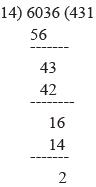Required number = (14 – 2) = 12

QUESTION: 5

If there are two odd numbers say x and y, then which of the following expression is even?

Solution:

The sum of two odd numbers is even. So, x + y is even.

QUESTION: 6

What is the value of given number sentence? 144 × 362 ÷ 432 =?

Solution:

144 × 362 ÷ 432 = 144 × 1296 ÷ 432
= 144 × 3  = 432

QUESTION: 7

Solve the following:
43729 × 999 = ?

Solution:

43729 × 9999 = 43729 × (1000 – 1)
= 43729 × 1000 – 43729 × 1
= 43729000 – 43729
= 436 85271

QUESTION: 8

Which of the following is the smallest whole number if the number 418 * 342 is completely divisible by 3?

Solution:

Sum of digits = (4 + 1 + 8 + x + 3 + 4 + 2) =
(22 + x), which must be divisible by 3.
∴ x = 2

QUESTION: 9

Choose the correct number that can replace the question mark (?).
(?) – 18637 – 23994 = 9977

Solution:

(?) – 18637 – 23994 = 9977
(?) = 9977 + 18637 + 23994
= 52608

QUESTION: 10

Which of the following is the largest 3-digit prime number?

Solution:

997 is the largest 3- digit prime number.

QUESTION: 11

Choose the number which is not prime number?

Solution:

627 is divisible by 3. Hence it is not a prime number.

QUESTION: 12

How many prime numbers exist between 400 and 500?

Solution:

Prime numbers between 400 and 500 are:
401    409     419    421    431    433    439    443
449    457    461    463    467    479    487    491
499

QUESTION: 13

The sum of 65 natural number is:

Solution:

Sum of all the first n natural numbers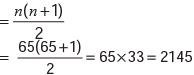QUESTION: 14

How many prime numbers are less than 40?

Solution:

Prime numbers less than 40 are:
2      3      5      7     11     13     17     19     23    29     31     37

QUESTION: 15

The algebraic expression 73n– 42n has a factor:

Solution:

73n – 42n = (73) n – (42) n
= (343)n – (16)n
Thus 343 – 16 is a factor i.e. 327 is a factor of 73n – 42n

QUESTION: 16

Which of the following rational numbers should be subtracted from 4/5to get 0.5?

Solution:

4/5 = 0.8
Hence, required number = 0.8 – 0.50  = 0.3

QUESTION: 17

The additive inverse of 7.22 is added to the additive identity of 3.6. What is the sum obtained?

Solution:

Additive inverse of 7.22 = – 7.22
Additive identity of 3.6   = 0
Hence, sum of – 7.22 and 0 = – 7.22

QUESTION: 18

The multiplicative inverse of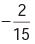is added to the additive inverse of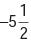. Find the sum.

Solution:

Multiplicative inverse of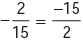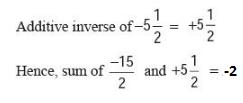QUESTION: 19

1/5 is first taken away from 1.5 and then 6/5 is added to the result. Which of the following is the new rational number obtained?

Solution: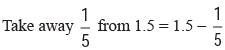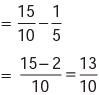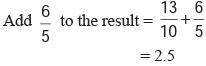QUESTION: 20

The rational number 3.78 is increased by 1/5 and then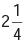is subtracted from the result. Find the new rational number.

Solution:

[{(3.78 + 1/5) - 2} - 1/4]

=> {(3.98) - 2} - 1/4

=> 1.98 - 1/4

=> 1.73